## research papersJOURNAL OFSYNCHROTRONRADIATION
ISSN: 1600-5775
Volume 6| Part 5| September 1999| Pages 1007-1015

## X-ray charge density study of p-amino-p′-nitrobi­phenyl at 20 K using a CCD area detector and synchrotron radiation: a very large dipole moment enhancement in the solid state

aChemistry Department, State University of New York at Buffalo, Buffalo, NY 14260-3000, USA
*Correspondence e-mail: coppens@acsu.buffalo.edu

(Received 11 January 1999; accepted 13 April 1999)

Accurate 20 K intensity data were collected on a crystal of p-amino-p′-nitrobiphenyl [a = 24.348(1), b = 5.802 (1), c = 7.158 (1) Å, α = β = γ = 90°, space group Pca21] at the SUNY X3A1 beamline at the National Synchrotron Light Source, using a CCD area detector and a DISPLEX cryostat. The sharp vertical profile of the synchrotron beam, combined with a slight instability of the cryostat, necessitates a φ-dependent correction, made possible by a large redundancy in the data set, the average multiplicity of the measurements being 8.02. In the multipole refinement, net atomic charges were constrained to those from a κ-refinement, corresponding to a more local definition of the pseudoatoms in the crystal. Both localized and unrestricted refinements show a very large (more than threefold) enhancement of the dipole moment in the solid state compared with that of the isolated molecule.

### 1. Introduction

With the advance of very bright third-generation synchrotron sources, analysis of the structure of excited states of molecules is becoming a realistic possibility. It is therefore necessary to identify systems suitable for such studies (Coppens et al., 1998). One area of interest is intramolecular electron transfer from electron-donor to electron-acceptor groups, leading to a highly dipolar charge-separated state. Such charge-separated states occur in the initial stages of photosynthesis, with the charge-separated state being stabilized by ionic interactions (Jortner & Pullman, 1990). The charge-separated species is both a reducing and an oxidizing agent.

p-Amino-p′-nitrobiphenyl (PANB) (4-4′-aminonitro-biphenyl) has a charge-separated excited triplet state with a long lifetime (1.4 µs) in a solution in tetrahydrofurane at room temperature (Piotrowiak et al., 1995). The lifetime of the triplet state in the neat crystal is not yet known, but may be affected by exciton–exciton annihilation at higher concentrations. However, such interactions between excitons can be reduced by complexing the photo-active molecule with a photo-inert host (Zhang et al., 1999).

As may be expected for a charge-separated state, there is evidence that the excited state of PANB has a greatly enhanced dipole moment. Measurements of the polarization of the fluorescence and the spectral shift of the absorption and fluorescence bands indicate a dipole moment increase of 12–16 D upon excitation of PANB in solutions in benzene and dioxane (Czekalla et al., 1963).

Before such an increase can be analyzed by time-resolved diffraction measurements on crystals, a second effect has to be considered. The dipole moment of a molecule in a crystal is increased relative to that of the isolated molecule by induced polarization resulting from electrostatic interactions with the molecular environment. The effect can be quite pronounced. For 2-methyl-4-nitroaniline, for example, an increase from 8.2–8.4 D for the isolated molecule to ∼22–24 D for the molecule in the crystal has been reported, and qualitatively confirmed by a crystal-field effect calculation (Howard et al., 1992).

Though X-ray charge-density analysis is now rather routinely used to derive solid-state electrostatic moments, the moments from an aspherical-atom multipole refinement are sensitive to the details of the refinement procedure. As part of a critical evaluation of electrostatic moments from diffraction data (Abramov et al., 1999), and as a preliminary to subsequent time-resolved studies, we have performed an accurate 20 K charge density analysis of PANB, using 0.643 Å synchrotron radiation and a CCD area detector as the recording device. Specific difficulties associated with accurate data collection at a synchrotron source are discussed in some detail.

### 2. Experimental

PANB was prepared by nitration of 4-nitrobiphenyl with nitric acid as described by Gull & Turner (1929). 4-4′-Dinitrobiphenyl was isolated and reduced with sodium hydrosulfide following Idoux (1970) to give PANB, which was recrystallized from chloroform. Data were collected at the SUNY X3A1 beamline at the National Synchrotron Light Source, Brookhaven National Laboratory. A wavelength of 0.643 Å obtained with a sideways-reflecting curved Si(111) monochromator was used. A well faceted crystal with dimensions 0.14 × 0.10 × 0.03 mm was glued onto the tip of an amorphous carbon fiber, and mounted on the cold finger of the two-stage close-cycle helium DISPLEX CT211 cryostat. The cryostat was mounted on the φ-table of a HUBER D-511.1 four-circle diffractometer (Graafsma et al., 1991) equipped with a BRUKER SMART 1000 CCD area detector. A special anti-scattering' device (Darovsky et al., 1994) was mounted inside the chamber of the cryostat in order to reduce the scattering of the direct beam by the graphite walls of the vacuum chamber. All measurements were performed at 20(1) K. The detector surface was located at 4.7 cm from the crystal, which is the shortest distance allowed by the vacuum chamber.

PANB crystallizes in the orthorhombic system in the space group Pca21. Unit-cell parameters [a = 24.348 (1), b = 5.802 (1), c = 7.158 (1) Å, α = β = γ = 90° (20 K)] were determined using 900 reflections with I > 20σ(I) measured in the φ range from 0 to 270°. Four data sets were collected at four different 2θ settings of the detector arm (0, 30, 45 and 55°), labeled data sets 1, 2, 3 and 4, respectively. The data were collected by rotation of the φ axis from 0 to 360° with an interval of 0.3° per frame. In two cases the data collection was interrupted because of a beam dump. As a result, only the reflections from 0 to 318° and 0 to 276° were recorded for data sets 2 and 4, respectively. As the 2θ ranges overlapped considerably, this did not lead to any significant loss of unique reflections. The intensities were integrated with the SAINT software package (Siemens, 1996). During the integration, the orientation matrix was optimized after every 50 frames. The incident beam intensity was normalized based on the counts from a beam monitor placed after the beam-defining slits. No absorption correction was applied because of the small size of the specimen and the low absorption coefficient (μ ≃ 0.86 cm−1).

### 3. Data reduction

Initially, each of the four data sets was internally averaged with the program SORTAV (Blessing, 1997) using all measured reflections (Table 1). A number of reflections absent because of the beamstop shadow were eliminated from the list. Detailed analysis of the symmetry-equivalent reflections revealed a smooth dependence of the integrated intensities on the rotation angle φ.

 Table 1Data collection information
Data set 1 2 3 4 5
Temperature (K) 20 20 20 20 20
χ angle of the cryostat (°) 0 0 0 0 90
2θ angle of the detector (°) 0 30 45 55 12.5
φ rotation range (°) 0–360 0–318 0–360 0–276 0–360
sin θ/λ range (Å−1) 0.041–0.585 0.082–0.848 0.275–0.984 0.411–1.068 0.041–0.585
Total measured reflections 4106 8238 12718 11085 3115
Systematic absences 398 329 363 314 281
Reflections used for averaging 3708 7909 12355 10777 2835
Unique reflections 667 2163 3342 3180 722
N 5.1 3.7 3.7 3.4 3.9
R-merge§ before correction (%) 15.44 14.99 11.67 12.04 6.38
R-merge after correction (%) 7.85 7.00 6.73 5.97
†Data were not collected up to φ = 360° because of beam dump.
‡〈N〉 is an overall average measurement multiplicity.
§Rmerge defined as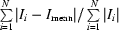, where N is the number of reflections in the data set.

For further analysis, the ratio Ii/Iav was plotted against the rotation angle φ for all independent reflections for which four or more equivalents were recorded, and for which Ii > 5σ(Ii). Here Ii is the intensity of the independent measurement (i = 1 . . . nmeas, where nmeas is the total number of symmetry-equivalent and repeated measurements) and Iav is the averaged intensity for a particular hkl. The resulting curves were found to have sinusoidal shapes with considerable amplitudes (Fig. 1). The intensity effect was traced to the very narrow vertical dimension of the synchrotron beam (Fig. 2), combined with a slight instability in the vertical direction of the horizontally mounted cryostat. Upon rotation of the cryostat around φ, a movement of the sample of ∼0.3 mm was observed, both optically and from the positions of the equatorial reflections of the carbon fiber on which the crystal was mounted. It could not be corrected by changing the centering of the sample. As is shown in Fig. 2, the plateau of the beam profile is only about 0.24 mm (vertical) × 0.48 mm (horizontal), thus, even a small sample motion can lead to significant intensity errors.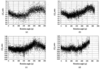Figure 1 Ratio Ii/Iav as a function of the rotation angle φ for data sets collected at (a) 0°, (b) 30°, (c) 45° and (d) 55° 2θ positions of the detector.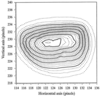Figure 2 Contour map of the direct beam intensity distribution. The solid line contour interval is 4000 counts, and that of the dotted line is 1000 counts. The zero contour line is omitted. Pixel size 120 µm.

Since the beam profile is broader in the horizontal direction, and forces due to the cryostat weight would be directed towards its base and thus be less effective, a data set collected with the cryostat axis vertical should be less susceptible to instabilities perpendicular to the cryostat axis. The vertical orientation of the cryostat, however, does not allow collection of high-order data, as in this orientation the detector arm cannot be moved to angles beyond ∼13° without sacrificing the short sample–detector distance. A vertical' data set (5) was collected with 2θdetector = 12.5°. As expected, this data set did not show the dependence of Ii/Iav from the rotation angle φ (Fig. 3), thus supporting the interpretation of the intensity effect.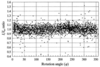Figure 3 Ratio Ii/Iav as a function of the rotation angle φ for data collected with the cryostat in the vertical position. 2θdetector = 12.5°.

As the vertical beam size is dictated by the synchrotron parameters and beamline optics, which cannot be easily changed, it was decided to apply an empirical correction to the intensity data. As the φ-dependence of the intensities appeared to be too complex to be described by a single function, the locally weighted regression scatter plot smoothing' method (LOWESS) (Chambers et al., 1983) was used to derive the correction. This robust technique is almost insensitive to outliers, in contrast to smoothing' (i.e. simple averaging over a range of adjacent points). The fraction of the total points used in the calculation of a point is defined as the smoothing factor. A weighted linear least-squares method is then used to fit a line to the neighboring points, with the weights assigned to each of the points being a function of the distance along φ of the points to the point being averaged. The final refined value at the point of averaging is calculated with weights based both on the φ distance and the deviation of each of the individual points to the first least-squares line. In Fig. 4the results of the LOWESS method are compared with simple smoothing results for data set 2, while the calculated correction curves for data sets 1, 3 and 4 are shown in Fig. 5. The corrected data are plotted against φ in Fig. 6.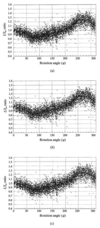Figure 4 Correction curves for data set 2 (2θdetector = 30°). (a) Averaging over the range of 4.4° (total 72 points), (b) averaging over the range of 9.91° (total 32 points), (c) locally weighted regression scatter plot smoothing' (LOWESS) method (smoothing factor of 20%).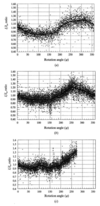Figure 5 Correction curves for data sets (a) 1, (b) 3 and (c) 4.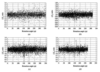Figure 6 Ii/Iav as a function of rotation angle (φ) after correction for data sets (a) 1, (b) 2, (c) 3 and (d) 4.

The corrected data were inter-scaled and averaged in program SORTAV (Blessing, 1997). Reflections that deviated more than 5σ from the maximum intensity within a set of equivalents were rejected before inter-subset scaling. This resulted in a total of 37578 reflections before averaging, with an average multiplicity of 8.2 reflections per unique reflection, and a final R-merge factor of 3.97%. 506 reflections were measured once, 396 reflections twice and 3274 reflections three or more times. Only the last group was used in the subsequent analysis.

### 4. Least-squares refinements

Structure analysis using SHELXS86 (Sheldrick, 1986) confirmed the previously reported structure (Graham et al., 1989). H atoms were located in a Fourier difference map, following full-matrix refinement with the program SHELXL97 (Sheldrick, 1997). Comparison of Fobs and Fcalc for the strong reflections showed extinction not to be a factor, as was expected given the small crystal size and the relatively short wavelength used. The residuals after the final cycle of refinement are given in Table 2. The ORTEP (Johnson, 1976) plot of a single molecule is shown in Fig. 7(a). The crystal consists of extended N—HO—N hydrogen-bonding sheets of molecules oriented perpendicular to the c axis, with each PANB molecule hydrogen-bonded to four other molecules (Fig. 7b, Table 51).

 Table 2Summary of least-squares refinements
 All refinements were performed with XD unless specified otherwise.
Conventional (IAM)
SHELXL97 XD High angle κ Pv restricted Unrestricted
Nref 3274 2615 1447 2615 2615 2615
Npar 184 184 144 34 255 267
Nref/Npar 17.8 14.2 10.0 76.9 10.3 9.8
RF (%) 3.72 3.11 1.82 2.99 1.72 1.69
wRF2 (%) 8.73 8.53 4.15 8.44 4.06 3.98
Goodness of fit 0.969 1.090 0.457 1.045 0.525 0.517
Max (Δ/σ) 1 × 10−3 6 × 10−7 3 × 10−12 8 × 10−3 2 × 10−3 0.1
Mean (Δ/σ) <1 × 10−4 2 × 10−10 5 × 10−15 2 × 10−6 5 × 10−7 6 × 10−6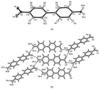Figure 7 (a) ORTEP plot of the PANB molecule. (b) The hydrogen-bonding network in a sheet of PANB molecules perpendicular to the c axis.

The aspherical atom multipole refinement was carried out using the package XD (Koritsanszky et al., 1997), and based on F. In total, 2615 reflections with Fobs > 3σ(Fobs) were used in the refinements. H atoms were positioned at a distance of 1.08 Å from the C atom along the C—H bond vector and a distance of 1.01 Å from N along the N—H bond vector. These distances correspond to the averaged C—H bond distance in aromatic rings and the averaged N—H bond distance in the X2—N—H group (International Tables for Crystallography, 1995). The positional and anisotropic thermal parameters were refined for all non-H atoms. For the H atoms the riding' model, which equates the shifts to those of the atom the H atom is bonded to, was applied. Isotropic temperature factors for the H atoms were refined.

As noted above, one of the aims of the current study is the determination of the dipole moment of PANB in the solid state. However, the standard multipole model (Coppens, 1997) may not produce truly localized atoms, because of a redistribution of the charge between atoms through increased population of the multipoles. This violates Kurki-Suonio's requirement of locality, which states that density at a point should be assigned to a center in the proximity of that point (Kurki-Suonio, 1968; Kurki-Suonio & Salmo, 1971). A modified procedure (Abramov et al., 1999) was therefore adopted. In the first stage of the procedure, positional parameters and anisotropic thermal parameters of the non-H atoms were determined by high-order refinement (sin θ/λ > 0.7 Å−1). In the second stage, the scale factor, monopole population parameters (Pv) and radial κ (valence-shell expansion–contraction) parameters of the non-H atoms were refined, with the κ parameters of the H atoms (but not Pv for H) fixed at 1.2. In this κ-refinement (Coppens et al., 1979; Coppens, 1997), the molecule was constrained to have mirror symmetry along its long axis, while C—H and N—H H atoms were treated as equivalent in each group.

In the final stage, the Hansen–Coppens electron density model (Hansen & Coppens, 1978) was applied with the crucial restriction that monopole populations were fixed at those from the κ-refinement, thus imposing locality on the atomic charge distribution. In order to reduce the number of parameters, the multipole coefficients of the non-H atoms were constrained to obey local mirror-plane symmetry (m), the mirror plane being defined as the plane through the atom and two adjacent atoms, while H atoms were given cylindrical symmetry. Additional constraints corresponding to the (mm) symmetry were imposed on N1, C1, C4, C7, C10 and N2, located on the long axis of the molecule. No constraints were applied to the positional or thermal parameters of the non-H atoms. For the C, N and O atoms all symmetry-allowed population coefficients up to, and including, the octupoles were refined, while for H atoms the dipole and quadrupole populations allowed by the local cylindrical symmetry were included. Local coordinate systems in which the multipoles are defined are shown in Fig. 8. Radial parameters κ and κ′ were refined for all non-H atoms but were again fixed at 1.2 for the H atoms.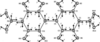Figure 8 Local coordinate systems used to define the atom-centered multipolar functions. R and L denote right- and left-hand systems, respectively.

The results of the different least-squares refinements are summarized in Table 2, while a final residual density map in the plane through atoms C6, C8 and C11 is shown in Fig. 9. Dynamic and static deformation density maps in the same plane are reproduced in Fig. 10. Results of the Hirshfeld rigid-bond test (Hirshfeld, 1976) (Table 61) show the advantages of very low temperature data collection. While the maximum discrepancy is 0.0013 Å2 for the spherical atom refinements, it is only 0.0005 Å2 for the final multipole refinement. Such discrepancies are comparable with the estimated standard deviations of the diagonal components Uij of the mean-square displacement amplitudes of non-H atoms, which range from 0.0001 to 0.0004 Å2. Monopole population (Pv) and radial parameters κ and κ′ for different refinements are given in Table 7,1 while net atomic charges derived from the monopole populations are listed in Table 3. Final observed and calculated structure factors are listed in Table 8.1

 Table 3Net atomic charges of the atoms in the PANB molecule from experimental and theoretical data
 Experimental values are based on monopole populations; theoretical values according to Mulliken population analysis. Chemically constrained atoms are indicated by an = sign.
Atoms Pv restricted multipole refinement Unconstrained multipole refinement HF/6-311G** B3LYP/6-311G**
O1 −0.52 −0.58 −0.39 −0.28
O2 −0.52 = O1 −0.58 = O1 −0.39 −0.28
N1 −0.71 −0.41 −0.28 −0.49
N2 +0.22 −0.64 +0.33 +0.16
C1 +0.72 +0.88 +0.24 +0.18
C2 +0.01 −0.11 −0.11 −0.10
C3 −0.24 +0.23 −0.05 −0.05
C4 +0.01 +0.26 −0.09 −0.08
C5 −0.24 = C3 +0.23 = C3 −0.06 −0.06
C6 +0.01 = C2 −0.11 = C2 −0.11 −0.09
C7 +0.46 +0.02 +0.03 −0.03
C8 0.00 +0.21 −0.12 −0.08
C9 −0.29 −0.55 −0.01 −0.06
C10 +0.12 +0.28 +0.08 +0.13
C11 −0.29 = C9 −0.55 = C9 −0.01 −0.06
C12 0.00 = C8 +0.21 = C8 −0.11 −0.07
H1A +0.31 +0.19 +0.23 +0.22
H1B +0.31 = H1A +0.19 = H1A +0.23 +0.22
H2 +0.08 +0.11 +0.09 +0.09
H3 +0.08 = H2 +0.11 = H2 +0.10 +0.09
H5 +0.08 = H2 +0.11 = H2 +0.10 +0.09
H6 +0.08 = H2 +0.11 = H2 +0.09 +0.09
H8 +0.08 = H2 +0.11 = H2 +0.10 +0.10
H9 +0.08 = H2 +0.11 = H2 +0.15 +0.13
H11 +0.08 = H2 +0.11 = H2 +0.15 +0.13
H12 +0.08 = H2 +0.11 = H2 +0.10 +0.10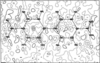Figure 9 Residual density map in the plane of atoms C11—C6—C8 after restricted Pv multipole refinement. Positive, negative and zero contours are represented by solid, dashed and dotted lines, respectively. The contour intervals are at 0.05 e Å−3. The maximum positive density in this plane is 0.13 e Å−3, the minimum negative density is −0.11 e Å−3.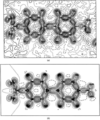Figure 10 Dynamic (a) and static (b) deformation density maps in the plane of atoms C11—C6—C8 after restricted multipole refinement. Positive, negative and zero contours are represented by solid, dashed and dotted lines, respectively. Contour intervals are 0.05 and 0.1 e Å−3 for (a) and (b), respectively.

### 5. Discussion

Quite drastic differences are found between the results of the restricted and unrestricted refinements (Table 3and Table 71). In particular, the N atom of the NO2 group, which is negatively charged in the unrestricted refinement (−0.64e), becomes positive (+0.22e) when the modified refinement procedure is applied. In a parallel study we have found that an analogous difference occurs for the carboxylic C atom in DL-histidine when the restricted procedure is adopted (Abramov et al., 1999). In PANB the change is associated with a slight contraction of the N valence shell, with κ changing from 0.971 to 1.018. In general, the modified procedure leads to net atomic charges, which are much closer to those from theory, and intuitively acceptable. For example, the negative charges on the O atoms of the nitro group would commonly lead to a positive charge on the central atom of the group.

Molecular dipole moments according to the different refinements are summarized and compared with the ab initio results for the isolated molecule in Table 4. The calculations were carried out at the Hartree–Fock (HF) and density-functional theory (DFT) levels using a 6-311G** basis set, which includes polarization functions on all atoms, giving a total of 18 and 6 basis functions on non-H and H atoms, respectively. Net atomic charges from a Mulliken population analysis are included in Table 3.

 Table 4Dipole moments (μ) for PANB
μ (Debye)
Crystal structure
κ refinement 36.0
Pv restricted multipole refinement 37.3
Unrestricted multipole refinement 73.5
Single molecule
HF/6-311G** 9.1
B3LYP/6-311G** 9.2

It is well known that the moments for a molecule in a solid are generally larger than those calculated theoretically for isolated molecules (see, for example, Gatti et al., 1994, 1995), in agreement with theoretical results for molecules in solution (Gao & Xia, 1992; Gao, 1996). The effect is especially pronounced for molecules with conjugated double bonds in polar solvents (Gao, 1997). For PANB, all refinements indicate a very large increase of the dipole moment upon crystallization. The polar structure of the sheets (Fig. 7b), in which molecules are lined up and linked by hydrogen-bonding between the terminal NH2 donor and the NO2 acceptor groups, is favorable to induced polarization. Given the length of the PANB molecule, this leads to a very large increase in the dipole.

A further discussion on the relative values of solid-state and isolated molecule dipole moments for this and other molecules will be published elsewhere.

### 6. Conclusions

Our experience demonstrates the importance of sample and beam stability when using very low divergent synchrotron sources. The correction technique developed in this study is only applicable when stability deviations are small, and then only to crystals belonging to the more symmetric space groups. It is quite possible that He gas-flow systems, now becoming available (Hardie et al., 1998), will alleviate the problems encountered.

The very large increase in the solid-state dipole moment relative to the isolated molecule value illustrates that theoretical quantities calculated for isolated molecules cannot be transferred blindly to molecular crystals. This is especially pertinent for the calculation of electrostatic contributions to intermolecular interactions.

### Footnotes

1Tables 5, 6, 7 and 8 have been deposited and are available from the IUCR electronic archives (Reference: HT2005 ). Services for accessing these data are described at the back of the journal.

### Acknowledgements

Support of this work by the National Science Foundation (CHE9615586) is gratefully acknowledged. The SUNY X3 beamline at NSLS is supported by the Division of Basic Energy Sciences of the US Department of Energy (DE-FG02-86ER45231). Research was carried out in part at the National Synchrotron Light Source at Brookhaven National Laboratory which is supported by the US Department of Energy, Division of Materials Sciences and Division of Chemical Sciences.

### ReferencesJOURNAL OFSYNCHROTRONRADIATION